## Example Questions

← Previous 1

### Example Question #8 : How To Find The Slope Of A Line

What is the slope of line 3 = 8y - 4x?

2

0.5

-0.5

-2

0.5

Explanation:

Solve equation for y. y=mx+b, where m is the slope

### Example Question #1 : How To Find The Slope Of A Line

If 2x – 4y = 10, what is the slope of the line?

0.5

–5/2

–0.5

2

–2

0.5

Explanation:

First put the equation into slope-intercept form, solving for y: 2x – 4y = 10 → –4y = –2x + 10 → y = 1/2*x – 5/2. So the slope is 1/2.

### Example Question #1 : How To Find The Slope Of A Line

What is the slope of the line with equation 4x – 16y = 24?

1/2

–1/8

1/8

1/4

–1/4

1/4

Explanation:

The equation of a line is:

y = mx + b, where m is the slope

4x – 16y = 24

–16y = –4x + 24

y = (–4x)/(–16) + 24/(–16)

y = (1/4)x – 1.5

Slope = 1/4

### Example Question #2 : How To Find The Slope Of A Line

What is the slope of a line which passes through coordinates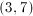and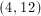?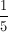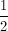Explanation:

Slope is found by dividing the difference in the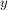-coordinates by the difference in the-coordinates.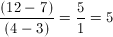### Example Question #3 : How To Find The Slope Of A Line

What is the slope of the line represented by the equation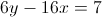?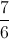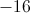Explanation:

To rearrange the equation into a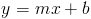format, you want to isolate the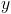so that it is the sole variable, without a coefficient, on one side of the equation.

First, add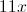to both sides to get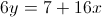.

Then, divide both sides by 6 to get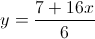.

If you divide each part of the numerator by 6, you get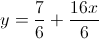. This is in a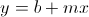form, and the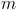is equal to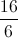, which is reduced down to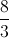for the correct answer.

### Example Question #2 : How To Find The Slope Of A Line

What is the slope of the given linear equation?

2x + 4y = -7

-1/2

-7/2

-2

1/2

-1/2

Explanation:

We can convert the given equation into slope-intercept form, y=mx+b, where m is the slope. We get y = (-1/2)x + (-7/2)

### Example Question #91 : Algebra

What is the slope of the line: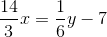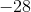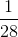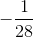Explanation:

First put the question in slope intercept form (y = mx + b):

(1/6)y = (14/3)x  7 =>

y = 6(14/3)x  7

y = 28x  7.

The slope is 28.

### Example Question #4 : How To Find The Slope Of A Line

What is the slope of a line that passes though the coordinates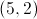and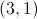?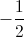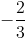Explanation:

The slope is equal to the difference between the y-coordinates divided by the difference between the x-coordinates.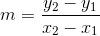Use the give points in this formula to calculate the slope.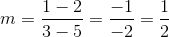### Example Question #1 : How To Find The Slope Of A Line

What is the slope of a line running through points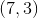and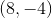?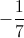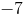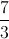Explanation:

The slope is equal to the difference between the y-coordinates divided by the difference between the x-coordinates.Use the give points in this formula to calculate the slope.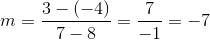### Example Question #8 : How To Find The Slope Of A Line

What is the slope of the line defined as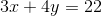?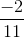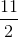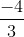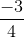Explanation:To calculate the slope of a line from an equation of the line, the easiest way to proceed is to solve it for.  This will put it into the format, making it very easy to find the slope.  For our equation, it is: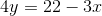or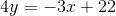Next you merely need to divide by: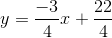Thus, the slope is← Previous 1